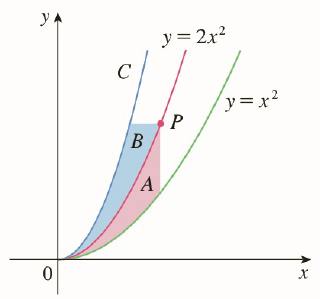Chapter 5.P, Problem 9P

Chapter
Section
Textbook Problem

# The figure shows a curve C with the property that, for every point P on the middle curve y = 2 x 2 , the areas A and B are equal. Find an equation for C.To determine

To find:

Equation for C

Explanation

1) Concept:

Construct integrals for area A and B, and then find the equation of curve C.

2) Calculation:

As point P lies on the curve y=2x2, with x=a, the y  co-ordinate of point P is 2a2.

So, let coordinates of point P be (a, 2a2).

To calculate the area A:

It is the area between curve y=2x2 and y=x2, between x=0 and x=a. So

A=0a2x2-x2dx

A=0ax2dx

A=x330a

A=a33

To calculate area B: It is the area between curves C and y=2x2 where y lies between 0 and a2.

It lies in the first quadrant.

Solve y=2x2 for x.

So,

x= fy=y2

Consider the equation for curve C as x=C(y).

So the area between curves is given by,

B=02a2y2-C(y)dy

B=43y23202a2-02a2Cydy

B=43a3-02

### Still sussing out bartleby?

Check out a sample textbook solution.

See a sample solution

#### The Solution to Your Study Problems

Bartleby provides explanations to thousands of textbook problems written by our experts, many with advanced degrees!

Get Started

#### Find y if y = ln(x2 + y2).

Single Variable Calculus: Early Transcendentals, Volume I

#### Simplify the expressions in Exercises 97106. x3/2x5/2

Finite Mathematics and Applied Calculus (MindTap Course List)

#### 6x2(3x2+2)+14(x+2)

Applied Calculus for the Managerial, Life, and Social Sciences: A Brief Approach

#### Find the perimeter of the square shown.

Elementary Geometry For College Students, 7e

#### sec1(23)= a) 3 b) 3 c) 6 d) 6

Study Guide for Stewart's Single Variable Calculus: Early Transcendentals, 8th